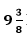Guru

# Two water taps together can fill a tank in NCERT Solutions for Class 10 Chapter 4- 1 hours. The tap of larger diameter takes 10 hours less than the smaller one to fill the tank separately. Find the time in which each tap can separately fill the tank. Q.9

• 0

I want the simplest and easiest way to solve this question. This theoretical question is very important for board exams so please help for solving this question  Two water taps together can fill a tank in NCERT Solutions for Class 10 Chapter 4- 1 hours. The tap of larger diameter takes 10 hours less than the smaller one to fill the tank separately. Find the time in which each tap can separately fill the tank.

Share

1. Let the time taken by the smaller pipe to fill the tank = x hr.

Time taken by the larger pipe = (x – 10) hr

Part of tank filled by smaller pipe in 1 hour = 1/x

Part of tank filled by larger pipe in 1 hour = 1/(– 10)

As given, the tank can be filled in= 75/8 hours by both the pipes together.

Therefore,

1/x + 1/x-10 = 8/75

x-10+x/x(x-10) = 8/75

⇒ 2x-10/x(x-10) = 8/75

⇒ 75(2x – 10) = 8x2 – 80x

⇒ 150x – 750 = 8x2 – 80x

⇒ 8x2 – 230x +750 = 0

⇒ 8x2 – 200x – 30x + 750 = 0

⇒ 8x(x – 25) -30(x – 25) = 0

⇒ (x – 25)(8x -30) = 0

⇒ x = 25, 30/8

Time taken by the smaller pipe cannot be 30/8 = 3.75 hours, as the time taken by the larger pipe will become negative, which is logically not possible.

Therefore, time taken individually by the smaller pipe and the larger pipe will be 25 and 25 – 10 =15 hours respectively.

• 0